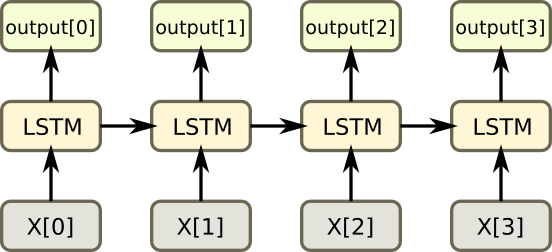# Recurrent Neural Network(RNN) Implementation

Posted by : at

Category :I heard about RNN for a long time, and have learned the concept several times, but until yesterday, I can’t implement any useful code to solve my own problem.

So I checked some tutorial. The most basic one is applying RNN to the MNIST dataset. The sample code is from sentdex’s video tutorial:

import tensorflow as tf
from tensorflow.examples.tutorials.mnist import input_data
from tensorflow.python.ops import rnn, rnn_cell
mnist = input_data.read_data_sets("/tmp/data/", one_hot = True)

hm_epochs = 3
n_classes = 10
batch_size = 128
chunk_size = 28
n_chunks = 28
rnn_size = 128

x = tf.placeholder('float', [None, n_chunks,chunk_size])
y = tf.placeholder('float')

def recurrent_neural_network(x):
layer = {'weights':tf.Variable(tf.random_normal([rnn_size,n_classes])),
'biases':tf.Variable(tf.random_normal([n_classes]))}

x = tf.transpose(x, [1,0,2])
x = tf.reshape(x, [-1, chunk_size])
x = tf.split(0, n_chunks, x)

lstm_cell = rnn_cell.BasicLSTMCell(rnn_size,state_is_tuple=True)
outputs, states = rnn.rnn(lstm_cell, x, dtype=tf.float32)
output = tf.matmul(outputs[-1],layer['weights']) + layer['biases']

return output

def train_neural_network(x):
prediction = recurrent_neural_network(x)
cost = tf.reduce_mean( tf.nn.softmax_cross_entropy_with_logits(prediction,y) )

with tf.Session() as sess:
sess.run(tf.initialize_all_variables())

for epoch in range(hm_epochs):
epoch_loss = 0
for _ in range(int(mnist.train.num_examples/batch_size)):
epoch_x, epoch_y = mnist.train.next_batch(batch_size)
epoch_x = epoch_x.reshape((batch_size,n_chunks,chunk_size))

_, c = sess.run([optimizer, cost], feed_dict={x: epoch_x, y: epoch_y})
epoch_loss += c

print('Epoch', epoch, 'completed out of',hm_epochs,'loss:',epoch_loss)

correct = tf.equal(tf.argmax(prediction, 1), tf.argmax(y, 1))

accuracy = tf.reduce_mean(tf.cast(correct, 'float'))
print('Accuracy:',accuracy.eval({x:mnist.test.images.reshape((-1, n_chunks, chunk_size)), y:mnist.test.labels}))

train_neural_network(x)


I suggest you to watch sentdex’s video tutorial first, and if you are not confident with what’s going on in the function recurrent_neural_network, you can go on read this article.

Let’s take a close look at this function:

def recurrent_neural_network(x):
layer = {'weights':tf.Variable(tf.random_normal([rnn_size,n_classes])),
'biases':tf.Variable(tf.random_normal([n_classes]))}

x = tf.transpose(x, [1,0,2])
x = tf.reshape(x, [-1, chunk_size])
x = tf.split(0, n_chunks, x)

lstm_cell = rnn_cell.BasicLSTMCell(rnn_size,state_is_tuple=True)
outputs, states = rnn.rnn(lstm_cell, x, dtype=tf.float32)
output = tf.matmul(outputs[-1],layer['weights']) + layer['biases']

return output


In the 1st line, we define variables for weights and biases. That’s common in any other neural network. If you don’t understand this line, you should go back and learn what is a neural network.

Next, we make some tricks to the input X. Tensorflow cannot output the value right away, as it define the whole model first and run later, so we can use numpy to mimik this tricks to see what happened.

import numpy as np
#First we define a small tensor to observe
x = np.arange(24).reshape(2,4,3)
print(x)
#Then do the transepose
x = x.transpose([1,0,2])
print(x)
#And do the reshape
x = x.reshape(-1,3)
print(x)
#And do the split
x = np.split(x, 4)
print(x)


the outcome should be like this (I pretty the output a little):

[[[ 0  1  2]
[ 3  4  5]
[ 6  7  8]
[ 9 10 11]]
[[12 13 14]
[15 16 17]
[18 19 20]
[21 22 23]]]

[[[ 0  1  2]
[12 13 14]]
[[ 3  4  5]
[15 16 17]]
[[ 6  7  8]
[18 19 20]]
[[ 9 10 11]
[21 22 23]]]

[[ 0  1  2]
[12 13 14]
[ 3  4  5]
[15 16 17]
[ 6  7  8]
[18 19 20]
[ 9 10 11]
[21 22 23]]

[array([[ 0,  1,  2],
[12, 13, 14]]), array([[ 3,  4,  5],
[15, 16, 17]]), array([[ 6,  7,  8],
[18, 19, 20]]), array([[ 9, 10, 11],
[21, 22, 23]])]


OK. We can see that, we finally have a list, which contains 4 elemnts. The 1st elements contains the 1st lines of the origin images. The 2nd contains the 2nd lines of origin images.

Now the final list is the input of the RNN.

After define a BasicLSTMCell cell, the next line is the key RNN implementation.

outputs, states = rnn.rnn(lstm_cell, x, dtype=tf.float32)


Let take a look at the source code of rnn.rnn on github, the programmer said that, the simplest form of RNN network generated is:

  state = cell.zero_state(...)
outputs = []
for input_ in inputs:
output, state = cell(input_, state)
outputs.append(output)
return (outputs, state)


That’s good! The output is also a list, just like the input list.Every single LSTM cell has a layer, which contain 128(rnn_size) neurals. At first, we feed the LSTM cell with the first slide of our input list, which is happen to be the whole first origin image. The first line of first origin image goes to the first LSTM first. Because the rnn has 128(rnn_size) neurals, so it will output 128 numbers this time. And then the 2nd line of first origin image goes to the 2nd LSTM, until meets the final line, and get another 128 numbers output… Finally, we will input all images in this batch, and get a batch of result.

And it comes to the final line:

output = tf.matmul(outputs[-1],layer['weights']) + layer['biases']


We see that only the last element of output is used. Sometimes we use all of the outputs list, and sometimes we just use the last one. That is because the information is contained in the last output. Why? Because this cell is called Long-short Term Memory(LSTM). It is designed to remember the whole sequence!

Here is a very good tutoral of What is LSTM.